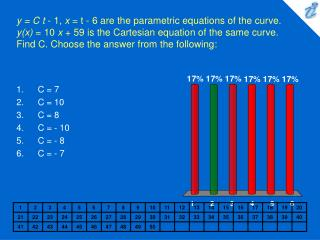DownloadDownload PresentationC = 7 C = 10 C = 8 C = - 10 C = - 8 C = - 7

# C = 7 C = 10 C = 8 C = - 10 C = - 8 C = - 7

Télécharger la présentation## C = 7 C = 10 C = 8 C = - 10 C = - 8 C = - 7

- - - - - - - - - - - - - - - - - - - - - - - - - - - E N D - - - - - - - - - - - - - - - - - - - - - - - - - - -
##### Presentation Transcript

1. y = C t - 1, x = t - 6 are the parametric equations of the curve. y(x) = 10 x + 59 is the Cartesian equation of the same curve. Find C. Choose the answer from the following: • C = 7 • C = 10 • C = 8 • C = - 10 • C = - 8 • C = - 7

2. 1. 2. 3. 4. Eliminate the parameter to find a Cartesian equation of the following curve: {image} , {image} Choose the answer from the following: • {image} • {image} • {image} • {image}

3. 1. 2. 3. Describe the motion of a particle with position (x, y) as t varies in the given interval {image} . {image} Choose the answer from the following: • Moves once clockwise along the circle {image} starting and ending at (0, 3). • Moves once clockwise along the ellipse {image} starting and ending at (0, 3). • Moves once counterclockwise along the ellipse {image} starting and ending at (0, 3).

4. 1. 2. 3. 4. Find parametric equations for the ellipse {image} Choose the answer from the following: • x = 7 sin t, y = 8 cos t, {image} • x = 7 cos t, y = 8 sin t, {image} • x = 7 sin t, y = 8 cos t, {image} • x = 7 cos t, y = 8 sin t, {image}

5. If a projectile is fired with an initial velocity of {image} meters per second at an angle {image} above the horizontal and air resistance is assumed to be negligible, then its position after t seconds is given by the parametric equations {image} , where g is acceleration of gravity ( {image} ). If a gun is fired with {image} and {image} , when will the bullet hit the ground? Choose the answer from the following: • t = 36 s • t = 72 s • t = 705.5 s